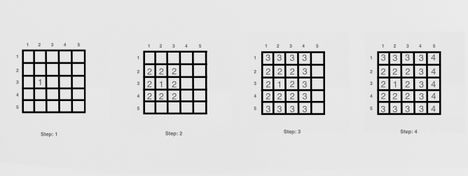# Matrix Resurrection

Limits 1s, 256 MB ·

We have a two-dimensional matrix consisting of $N$ rows and $M$ columns. The rows are numbered from $1$ to $N$ and the columns are numbered from $1$ to $M$. Two cells of the matrix will be called adjacent if they share a side vertically, horizontally, or diagonally.

Let’s say, we start with an empty matrix and fill it with integer numbers. To do that, first, we have to select a cell as the origin. We place integer $1$ in the cell. Then we repeat the following steps until all the cells of the matrix are filled. At each step, first we find the largest number in the matrix. Then, for all the cells containing that number, we fill the adjacent empty cells with the immediately larger number.

For example, let’s assume: $N = 5$ and $M = 5$.

Let the origin of the numbers be at $Row = 3$ and $Column = 2$.
In step: 1, we will place integer $1$ to the origin.
In step: 2, we will fill every adjacent cell of the origin with integer $2$.
In step: 3, we will fill all the empty cells that is adjacent to the cells that contain integer $2$ with integer $3$.
In step: 4, we will fill all the empty cells that is adjacent to the cells that contain integer $3$ with integer $4$.

We have filled the matrix with numbers. But the process will go on in the case of a larger matrix. The steps are illustrated in the figure below:This is an interactive problem. You will be given the size of the matrix. But the matrix will remain hidden. You can make at most $4$ queries. In each query, you have to print the row and column numbers of a cell. And you will receive what number is hidden in that cell.

After the interaction, you will have to print the whole matrix.

Interaction

The first line of input will consist of two integers $N$ and $M$ $(3 \leq N, M \leq 100)$ indicating the number of rows and the number of columns respectively.

To make a query print “0 R C“. Here $R$ and $C$ $(1 \leq R \leq N, 1 \leq C \leq M)$ are the rows and column numbers respectively. You are allowed to make at most $4$ queries of this type.

You will read an integer $X$ as a response to the query operation.

Here, $X$ is an integer situated in the $R^{th}$ row and $C^{th}$ column.

After you figure out the whole matrix, print integer $1$. Followed by $N$ lines. Each line will consist of $M$ space-separated integer numbers which represent the hidden matrix.

Also, keep in mind that any other output from your program will end up getting "Wrong Answer" as a verdict. If you do not perform the interactions properly, you may receive a “CPU Limit Exceeded” verdict or any other verdict.

Here is what a possible interaction would look like for the example matrix of the problem statement.

> 5 5
< 0 3 3
> 2
< 0 3 2
> 1
< 1
< 3 3 3 3 4
< 2 2 2 3 4
< 2 1 2 3 4
< 2 2 2 3 4
< 3 3 3 3 4


> indicates what your program reads and < indicates what your program writes. These symbols are here to make it easy to understand. You do not have to print such symbols from your program.

Note

Since this is an interactive problem, don’t forget to flush your output after printing each line. You may use fflush(stdout) for printf/cout in C/C++, import sys and sys.stdout.flush() in Python to flush the output. If you use some other programming language, consult it's documentation.